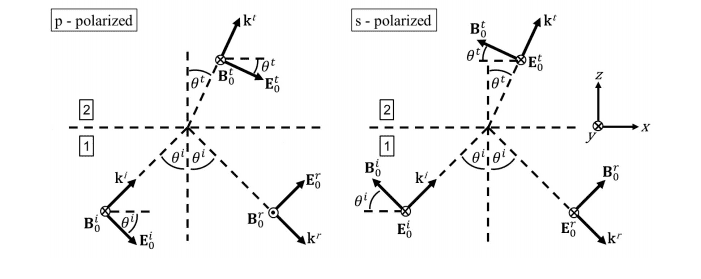# Confusion With Derivation of Fresnel Equations

• I
• bananabandana

#### bananabandanaOkay, so I'm working with the diagrams above. ##i## denotes incident, ##r## reflected, and ##t## transmitted.

-We're working in two HIL dielectrics. Incoming and outgoing waves are of form ##Aexp[i(\vec{k}\cdot\vec{r}- \omega t) ##. As I understand it, Maxwell's equations give four boundary conditions for this - Let:
• ##\vec{\hat{n}}## be a unit normal vector to the interface,
• ##\rho{SC}## is the surface free charge
• Subscript 1,2 refers to medium 1,2
Let's just look at the p-case:
• The parallel component of the ##\vec{E}## field must be continuous over the boundary.
• But the perpendicular component is also continuous over the boundary, since we know:
(1) $$\vec{\hat{n}} \cdot(\vec{D_{1}} -\vec{D_{2}}) = \rho_{SC}$$
and in a dielectric, ## \rho_{SC}=0##, and since the dielectric is HIL - ## |\vec{D}| = \epsilon|\vec{E}| ## , i.e:

(2) $$\vec{\hat{n}} \cdot \epsilon ( \vec{E_{1}} -\vec{E_{2}} ) =0$$

- For the wave to be continuous parallel to the boundary, we must have ##\theta_{incident} = \theta_{reflected} ##, as shown, and also that ## n_{1}sin(\theta^{i}) = n_{2} sin(\theta^{r}) ##. [Since the exponential terms must be equal]. So we can just work in terms of the amplitudes:

Then, for parallel continuity:

(3) $$(E_{0}^{i}+E_{0}^{r})cos \theta^{i} = E_{0}^{t}cos \theta^{t}$$

And for perpendicular continuity:

(4) $$(E_{0}^{r}-E_{0}^{i}) sin \theta^{i} = -E_{0}^{t} sin \theta^{t} \implies E_{0}^{t} sin \theta^{t} = (E_{0}^{i} -E_{0}^{r}) sin \theta^{i}$$

Solving these simultaneously, we arrive at the result:

(5) $$r = \frac{E_{0}^{r}}{E_{0}^{i}} = \frac{ cotan \theta^{t} - cotan \theta^{i} }{ cotan \theta^{i} + cotan \theta^{2} } = \frac{ cos\theta^{t} sin \theta^{i} -sin \theta^{t} cos \theta^{i} }{ cos \theta^{t} sin \theta^{i} + sin \theta^{i} cos \theta^{t}}$$

Except this is wrong, since if ## n_{1} sin \theta^{i} = n_{2} sin \theta^{t} ##, then we can rewrite (5) as:

(6) $$r= \frac{n_{2}cos \theta^{t} - n_{1} cos \theta^{i} }{ n^{2}cos\theta^{t}+n_{1}cos \theta^{i}}$$

Which is not the result my lecturer gets! Can someone explain where I made my mistake? Would be very grateful!